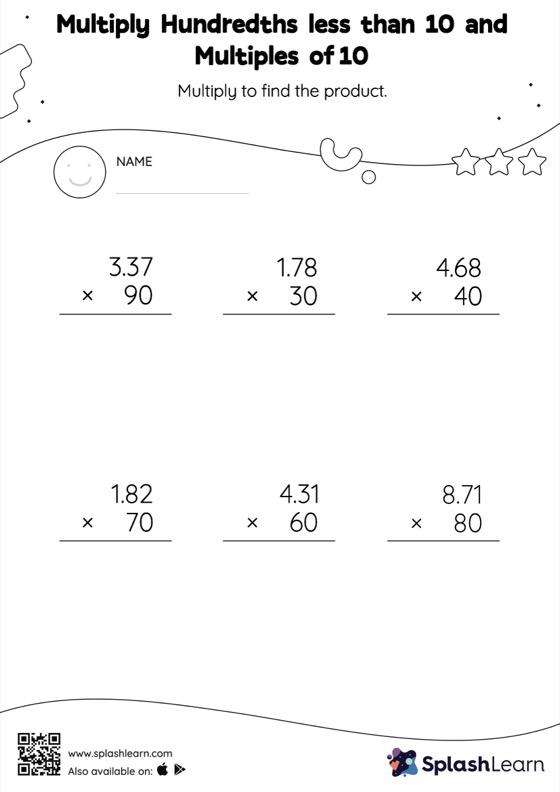# Multiply Hundredths less than 10 and Multiples of 10: Vertical Multiplication Worksheet

Home > Multiply Hundredths less than 10 and Multiples of 10: Vertical MultiplicationChallenge your young math wizards to solve this multiply hundredths less than 10 and multiples of 10 worksheet. When students multiply a decimal by a number ending in zeros, they first ignore the decimal point, multiply the numbers, and then add the decimal point in the product as many places from the right as the number of decimal digits in the original number.